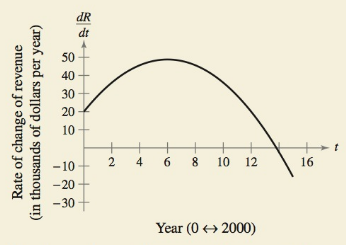Chapter 5.1, Problem 72E### Calculus: An Applied Approach (Min...

10th Edition
Ron Larson
ISBN: 9781305860919

#### Solutions

Chapter
Section### Calculus: An Applied Approach (Min...

10th Edition
Ron Larson
ISBN: 9781305860919
Textbook Problem
1 views

# HOW DO YOU SEE IT? The graph shows the rate of change of the revenue of a company from 2000 through 2015.(a) Approximate the rate of change of the revenue in 2012. Explain your reasoning.(b) Approximate the year when the revenue is maximum. Explain your reasoning.

(a)

To determine

The approximate rate of change of the revenue in 2012 with reason.

Explanation

Given Information:

The graph that shows the rate of change of the revenue of a company from 2000 through 2015 is shown below.

Consider the provided graph that shows the rate of change of the company is,

Draw a perpendicular line from the point 12 on the time axis. This line cuts the graph at some point...

(b)

To determine

The approximate year when the revenue is maximum with reason.

### Still sussing out bartleby?

Check out a sample textbook solution.

See a sample solution

#### The Solution to Your Study Problems

Bartleby provides explanations to thousands of textbook problems written by our experts, many with advanced degrees!

Get Started

#### Expand each expression in Exercises 122. (x2+x1)(2x+4)

Finite Mathematics and Applied Calculus (MindTap Course List)

#### In Exercises 1520, simplify the expression. 18. (2x3) (3x2) (16x1/2)

Applied Calculus for the Managerial, Life, and Social Sciences: A Brief Approach

#### List all of the subsets of {a, b, c, d}.

Mathematical Excursions (MindTap Course List)

#### True or False: If f′(c) = 0 and f″(c) < 0, then c is a local minimum.

Study Guide for Stewart's Single Variable Calculus: Early Transcendentals, 8th

#### True or False: If , then converge absolutely.

Study Guide for Stewart's Multivariable Calculus, 8th